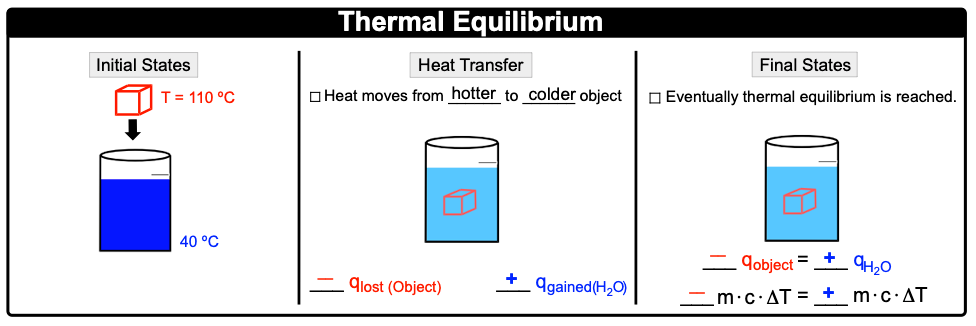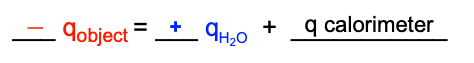Clutch Prep is now a part of Pearson
Ch.6 - Thermochemistry WorksheetSee all chapters

# Thermal Equilibrium

See all sections
Sections
Nature of Energy
Kinetic & Potential Energy
First Law of Thermodynamics
Internal Energy
Endothermic & Exothermic Reactions
Heat Capacity
Constant-Pressure Calorimetry
Constant-Volume Calorimetry
Thermal Equilibrium
Thermochemical Equations
Formation Equations
Enthalpy of Formation
Hess's Law
Enthalpy (IGNORE)

Thermal Equilibrium involves two substances that are in physical contact reaching the same final temperature over time.

###### Thermal Equilibrium Reactions

Concept #1: Thermal EquilibriumUnder non-ideal conditions, calorimeter absorbs some of the heat from the object.Example #1: If 50 g block of lead at 250 ºC is submerged in a solution at 90 ºC, the final temperature of the solution will be:

Practice: If 53.2 g Al at 120.0 ºC is placed in 110.0 g H2O at 90 ºC within an insulated container that absorbs a negligible amount of heat, what is the final temperature of the aluminum? The specific heat capacities of water and aluminum are 4.184 J/g ∙ ºC and 0.897 J/g ∙ ºC, respectively.